Professor of Computer Science @ Olin College

# ModSimPy Workshop

Last updated: December 13th, 2018

This notebook is part of my book: Modeling and Simulation in Python. See the source code on Github.

This is an interactive Jupyter Notebook that you can fork and start working right away. Click on the fork button on your right 👉.

In :
# Configure Jupyter so figures appear in the notebook
%matplotlib inline

# Configure Jupyter to display the assigned value after an assignment
%config InteractiveShell.ast_node_interactivity='last_expr_or_assign'

# import functions from the modsim.py module
from modsim import *

from pandas import read_html


### Functions from the previous chapter¶

In :
def plot_results(census, un, timeseries, title):
"""Plot the estimates and the model.

census: TimeSeries of population estimates
un: TimeSeries of population estimates
timeseries: TimeSeries of simulation results
title: string
"""
plot(census, ':', label='US Census')
plot(un, '--', label='UN DESA')
plot(timeseries, color='gray', label='model')

decorate(xlabel='Year',
ylabel='World population (billion)',
title=title)

In :
def run_simulation(system, update_func):
"""Simulate the system using any update function.

system: System object
update_func: function that computes the population next year

returns: TimeSeries
"""
results = TimeSeries()
results[system.t_0] = system.p_0

for t in linrange(system.t_0, system.t_end):
results[t+1] = update_func(results[t], t, system)

return results


### Reading the data¶

In :
filename = 'data/World_population_estimates.html'
tables = read_html(filename, header=0, index_col=0, decimal='M')
table2 = tables
table2.columns = ['census', 'prb', 'un', 'maddison',
'hyde', 'tanton', 'biraben', 'mj',
'thomlinson', 'durand', 'clark']

In :
un = table2.un / 1e9
census = table2.census / 1e9
plot(census, ':', label='US Census')
plot(un, '--', label='UN DESA')

decorate(xlabel='Year',
ylabel='World population (billion)',
title='Estimated world population')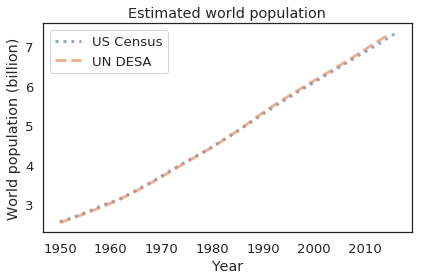### Running the quadratic model¶

Here's the update function for the quadratic growth model with parameters alpha and beta.

In :
def update_func_quad(pop, t, system):
"""Update population based on a quadratic model.

pop: current population in billions
t: what year it is
system: system object with model parameters
"""
net_growth = system.alpha * pop + system.beta * pop**2
return pop + net_growth


Extract the starting time and population.

In :
t_0 = get_first_label(census)
t_end = get_last_label(census)
p_0 = get_first_value(census)

Out:
2.557628654

Initialize the system object.

In :
system = System(t_0=t_0,
t_end=t_end,
p_0=p_0,
alpha=0.025,
beta=-0.0018)

Out:
values
t_0 1950.000000
t_end 2016.000000
p_0 2.557629
alpha 0.025000
beta -0.001800

Run the model and plot results.

In :
results = run_simulation(system, update_func_quad)
plot_results(census, un, results, 'Quadratic model')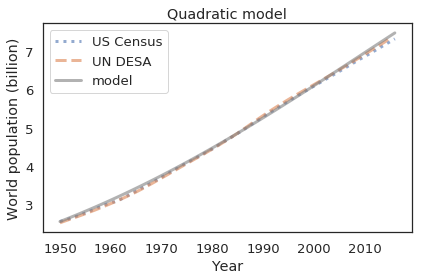### Generating projections¶

To generate projections, all we have to do is change t_end

In :
system.t_end = 2250
results = run_simulation(system, update_func_quad)
plot_results(census, un, results, 'World population projection')
savefig('figs/chap04-fig01.pdf')

Saving figure to file figs/chap04-fig01.pdf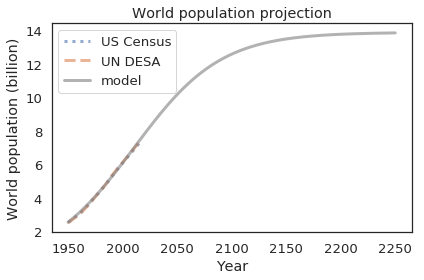The population in the model converges on the equilibrium population, -alpha/beta

In :
results[system.t_end]

Out:
13.856665141368708
In :
-system.alpha / system.beta

Out:
13.88888888888889

Exercise: What happens if we start with an initial population above the carrying capacity, like 20 billion? Run the model with initial populations between 1 and 20 billion, and plot the results on the same axes.

In [ ]:
# Solution goes here. Output preserved for guidance.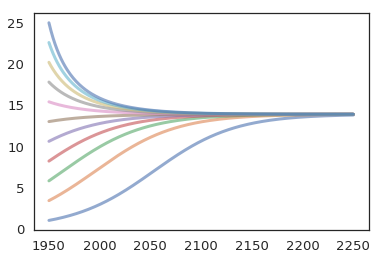### Comparing projections¶

We can compare the projection from our model with projections produced by people who know what they are doing.

In :
table3 = tables
table3.head()

Out:
United States Census Bureau (2015) Population Reference Bureau (1973-2015) United Nations Department of Economic and Social Affairs (2015)
Year
2016 7.334772e+09 NaN 7.432663e+09
2017 7.412779e+09 NaN NaN
2018 7.490428e+09 NaN NaN
2019 7.567403e+09 NaN NaN
2020 7.643402e+09 NaN 7.758157e+09

NaN is a special value that represents missing data, in this case because some agencies did not publish projections for some years.

In :
table3.columns = ['census', 'prb', 'un']


This function plots projections from the UN DESA and U.S. Census. It uses dropna to remove the NaN values from each series before plotting it.

In :
def plot_projections(table):
"""Plot world population projections.

table: DataFrame with columns 'un' and 'census'
"""
census_proj = table.census / 1e9
un_proj = table.un / 1e9

plot(census_proj.dropna(), 'b:', label='US Census')
plot(un_proj.dropna(), 'g--', label='UN DESA')


Run the model until 2100, which is as far as the other projections go.

In :
system = System(t_0=t_0,
t_end=2100,
p_0=p_0,
alpha=0.025,
beta=-0.0018)

Out:
values
t_0 1950.000000
t_end 2100.000000
p_0 2.557629
alpha 0.025000
beta -0.001800
In :
results = run_simulation(system, update_func_quad)

plot_results(census, un, results, 'World population projections')
plot_projections(table3)
savefig('figs/chap04-fig02.pdf')

Saving figure to file figs/chap04-fig02.pdf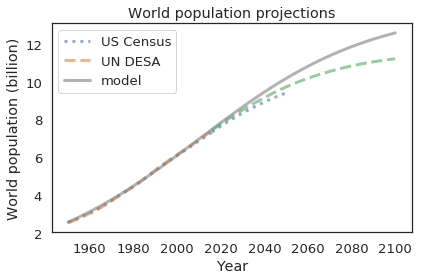People who know what they are doing expect the growth rate to decline more sharply than our model projects.

## Exercises¶

Optional exercise: The net growth rate of world population has been declining for several decades. That observation suggests one more way to generate projections, by extrapolating observed changes in growth rate.

The modsim library provides a function, compute_rel_diff, that computes relative differences of the elements in a sequence. It is a wrapper for the NumPy function ediff1d:

In :
%psource compute_rel_diff

def compute_rel_diff(seq):
xs = np.asarray(seq)
diff = np.ediff1d(xs, np.nan)
return diff / seq


Here's how we can use it to compute the relative differences in the census and un estimates:

In :
alpha_census = compute_rel_diff(census)
plot(alpha_census)

alpha_un = compute_rel_diff(un)
plot(alpha_un)

decorate(xlabel='Year', label='Net growth rate')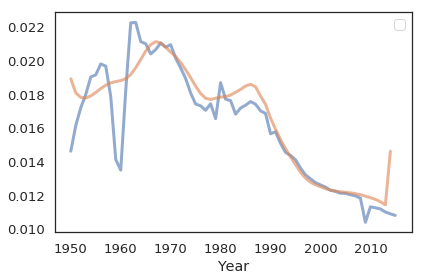Other than a bump around 1990, net growth rate has been declining roughly linearly since 1965. As an exercise, you can use this data to make a projection of world population until 2100.

1. Define a function, alpha_func, that takes t as a parameter and returns an estimate of the net growth rate at time t, based on a linear function alpha = intercept + slope * t. Choose values of slope and intercept to fit the observed net growth rates since 1965.

2. Call your function with a range of ts from 1960 to 2020 and plot the results.

3. Create a System object that includes alpha_func as a system variable.

4. Define an update function that uses alpha_func to compute the net growth rate at the given time t.

5. Test your update function with t_0 = 1960 and p_0 = census[t_0].

6. Run a simulation from 1960 to 2100 with your update function, and plot the results.

7. Compare your projections with those from the US Census and UN.

In [ ]:
# Solution goes here

In [ ]:
# Solution goes here

In [ ]:
# Solution goes here

In [ ]:
# Solution goes here

In [ ]:
# Solution goes here

In [ ]:
# Solution goes here

In [ ]:
# Solution goes here

In [ ]:
# Solution goes here


Related viewing: You might be interested in this video by Hans Rosling about the demographic changes we expect in this century.